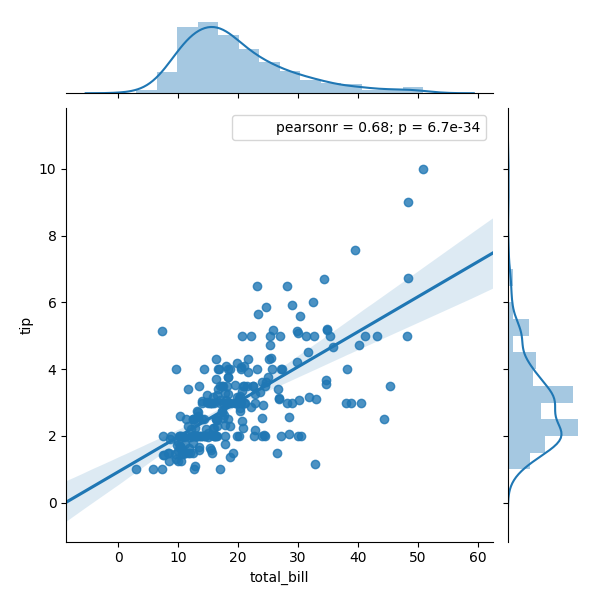Back to the main Index

## How to use ipython widgets to analyze pandas Dataframes¶

Pandas dataframes are very powerful and quite handy when one needs to analyze the results of multiple calculations or a large dataset. Several AbiPy objects (e.g robots) can construct dataframes and one can then use the pandas plot method for quick plots, or the seaborn package for more advanced visualizations.

Unfortunately, this approach becomes a bit tedious when we have to deal with several columns or when we need to customize the plot and we are not pandas/seaborn gurus.

For this reason, AbiPy provides a set of ipython widgets wrapping the Dataframe plot method and the seaborn API. The widgets are defined in abipy.display.seabornw for seaborn and abipy.display.pandasw for pandas:

In :
from __future__ import print_function, division, unicode_literals, absolute_import
%matplotlib notebook

import pandas as pd
import seaborn as sns # See https://seaborn.pydata.org/examples/index.html

# Import abipy widgets
import abipy.display.pandasw as pdw
import abipy.display.seabornw as snw


The idea is very simple. Instead of calling the seaborn API directly:

In :
tips = sns.load_dataset("tips")

sns.jointplot(x="total_bill", y="tip", data=tips, kind="reg");we call snw.joinplot to build a widget. The widget has controllers that allow us to select the variables to plot and change the options available for this kind of plot. Click the Run interact button to produce the figure:

In :
snw.joinplot(tips)

Out:
<function abipy.display.seabornw.joinplot.<locals>.sns_joinplot>

Use pdw.plot to create a widget wrapping the plot method of pandas Dataframe:

In :
iris = sns.load_dataset("iris")
pdw.plot(iris)

Out:
<function abipy.display.pandasw.plot.<locals>.plot_dataframe>

Now we can start to play with the seaborn API and our widgets:

In :
titanic = sns.load_dataset("titanic")

In :
snw.countplot(titanic)

Out:
<function abipy.display.seabornw.countplot.<locals>.sns_countplot>
In :
tips = sns.load_dataset("tips")

In :
snw.swarmplot(tips)

Out:
<function abipy.display.seabornw.swarmplot.<locals>.sns_swarmplot>
In :
snw.lmplot(tips)

Out:
<function abipy.display.seabornw.lmplot.<locals>.sns_lmplot>
In :
exercise = sns.load_dataset("exercise")

In :
snw.factorplot(exercise)

Out:
<function abipy.display.seabornw.factorplot.<locals>.sns_factorplot>
In :
snw.violinplot(tips)

Out:
<function abipy.display.seabornw.violinplot.<locals>.sns_violinplot>
In :
snw.stripplot(tips)

Out:
<function abipy.display.seabornw.stripplot.<locals>.sns_stripplot>
In :
snw.swarmplot(tips)

Out:
<function abipy.display.seabornw.swarmplot.<locals>.sns_swarmplot>
In :
snw.pointplot(tips)

Out:
<function abipy.display.seabornw.pointplot.<locals>.sns_pointplot>
In :
snw.barplot(tips)

Out:
<function abipy.display.seabornw.barplot.<locals>.sns_barplot>
In :
import numpy as np; np.random.seed(0)
uniform_data = np.random.rand(10, 12)
snw.heatmap(uniform_data)

Out:
<function abipy.display.seabornw.heatmap.<locals>.sns_heatmap>
In :
flights = sns.load_dataset("flights")
flights = flights.pivot("month", "year", "passengers")

In :
snw.clustermap(flights)

Out:
<function abipy.display.seabornw.clustermap.<locals>.sns_clustermap>
In :
# Generate a random dataset with strong simple effects and an interaction
import numpy as np
n = 80
rs = np.random.RandomState(11)
x1 = rs.randn(n)
x2 = x1 / 5 + rs.randn(n)
b0, b1, b2, b3 = .5, .25, -1, 2
y = b0  + b1 * x1 + b2 * x2 + b3 * x1 * x2 + rs.randn(n)
df = pd.DataFrame(np.c_[x1, x2, y], columns=["x1", "x2", "y"])

# Show a scatterplot of the predictors with the estimated model surface
snw.interactplot(df)

Out:
<function abipy.display.seabornw.interactplot.<locals>.sns_interactplot>

Back to the main Index

In [ ]: